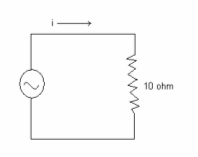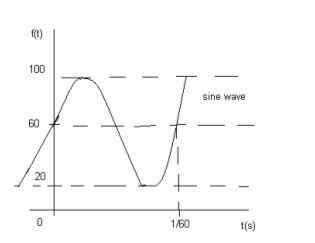# AC Fundamentals - Electronics Engineering test questions

## AC Fundamentals - Electronics Engineering test questions

(1) It is said that the two sinusoidal quantities are in phase quadrature when their phase difference is

(A) 30°
(B) 0°
(C) 90°
(D) 45°

(2) Consider a current sine wave whose rms value is 30 amperes. The equation for 25 cycles current sine wave will be

(A) 42.4 sin 50 π t
(B) 42.4 sin 25 π t
(C) 30 sin 50 π t
(D) 30 sin 50 π t

ANSWER: 42.4 sin 50 π t

(3) If E1 = A sin ωt and E2 = A sin (ωt – Φ), then

(A) E1 leads E2 by Φ
(B) E1 lags E2 by Φ/2
(C) E2 leads E1 by Φ
(D) E2 lags E1 by Φ

(4) _____________ is the rms value of rectangular voltage wave with and amplitude of 10 V.

(A) 11.2 V
(B) 5.2 V
(C) 7.7 V
(D) 10 V

(5) Consider 220 V, 50 Hz A.C. waveform. The form factor will be

(A) 1.5
(B) 1.11
(C) 1.14
(D) 0.85

(6) Let i = 100 sin ωt. How long will it take for the current to rise to 50 amperes if the frequency is 25 Hz?

(A) 1/30 sec
(B) 1/50 sec
(C) 1/1000 sec
(D) 1/100 sec

(7) Voltage V = 90 cos (ωt-161.5°). This may be represented as sine function by

(A) 90 sin (ωt – 18.5°)
(B) 90 sin (ωt + 18.5°)
(C) 90 sin (ωt + 71.5°)
(D) 90 sin (ωt – 71.5°)

ANSWER: 90 sin (ωt – 71.5°)

(8) The rms value of current i = 10 + 5 cos (628t + 30°) is

(A) 10.6 A
(B) 10 A
(C) 8 A
(D) 15 A

(9) The negative maximum of a cosine waveform occurs at

(A) 0°
(B) 270°
(C) 180°
(D) 90°

(10) Let us say that the current and voltage both are out of phase by 90°. The power will be

(A) Maximum
(B) Minimum
(C) Zero
(D) 1.1 VI

(11) Which one of the following has the least value of form factor?

(A) Sine wave
(B) Square wave
(C) Rectangular wave
(D) Triangular wave

(12) The frequency that has the longest period is

(A) 10 KHz
(B) 1 KHz
(C) 10 Hz
(D) 1 Hz

(13) The waveform that has the least rms value for the same peak value of voltage is

(A) Triangular wave
(B) Sine wave
(C) Square wave
(D) Full wave rectified sine wave

(14) Which of the following statement is false?

(A) The 480° vector will end up in second quadrant
(B) Every non-sinusoidal waveform has large number of harmonics
(C) A square wave has only even harmonics
(D) All of the above statements are true

ANSWER: All of the above statements are true

For questions 15 to 17 refer to the following data:

For equal intervals of time, a current has following steady values in amperes changing simultaneously from one to the next
0,10,20,30,20,10,0, -10, -20, -30, -20, -10, -0

(15) The rms value of current will be

(A) 10 A
(B) 17.8 A
(C) 178 A
(D) 100 A

(16) The form factor will be

(A) 1.187
(B) 1.0
(C) 1.100
(D) 1.11

(17) The peak factor of a wave is

(A) Rms value / average value
(B) Average value / rms value
(C) Maximum value / rms value
(D) Maximum value / average value

ANSWER: Maximum value / rms value

(18) For the voltage v = 12 sin (800 π t +0.125 π) V, the period will be

(A) 1.33 sec
(B) 133 sec
(C) 1.33 micro-second
(D) 1.33 milli-second

(19) If the load is same and the power factor of load is reduced, it will

(A) Draw less current
(B) Draw more current
(C) Draw less current but power will be less
(D) Nothing will happen

(20) The power factor of a load with Z = 36 Ω < -30° will be

(B) 0.866 lagging
(D) None of the above

(21) A certain square wave has a period of 4 ms. Its fundamental frequency will be

(A) 0 Hz
(B) 230 Hz
(C) 250 Hz
(D) 430 Hz

#### Discussion

• RE: AC Fundamentals - Electronics Engineering test questions -debakanta samal (06/24/15)
• it is an farbulus system &plat form to gain knowledge of ac fundamentalas
• AC Fundamentals - Electronics Engineering test questions -Slesha Shirkey (11/12/13)
• (22) A 100 Hz, 20 V waveform dissipates 10 watts in a 40 Ω resistor. The power dissipated will __________ when frequency is doubled

(A) Remain unchanged
(B) Increase
(C) Decrease
(D) Not be predicted

(23) The difference in period of two frequencies of 1MHz and 2 MHz is

(A) 0.5 milli-seconds
(B) 0.5 micro-seconds
(C) 1 milli-seconds
(D) 1 micro-seconds

For Questions 24 and 25 refer to data given below:

The supply voltage is 60 Hz with a maximum value of 160 V for the circuit shown below(24) The instantaneous current at π/4 radians will be

(A) 14.0 A
(B) 11.3 A
(C) 6.7 A
(D) 9.1 A

(25) The instantaneous power at π/4 radians will be

(A) 2.56 KW
(B) 1.1 KW
(C) 1.9 KW
(D) 5.5 KW

For Questions 26 and 27 refer to data given below:

v1 = 41 sin Θ and v2 = 33 sin (Φ + 20 °)

(26) (v1 + v2) will be equal to

(A) 80 sin (Θ - Φ - 20 °)
(B) 80 sin (Θ + Φ + 20 °)
(C) 77.8 sin (Φ - 8.3 °)
(D) 77.8 sin (Φ + 8.3 °)

ANSWER: 77.8 sin (Φ + 8.3 °)

(27) (v1 - v2) will be equal to

(A) 16 sin (Φ - 20 °)
(B) 16 sin 20 °
(C) 19.59 sin (Φ + 35 °)
(D) 19.59 sin (Φ - 35 °)

ANSWER: 19.59 sin (Φ - 35 °)

(28) The 4th harmonic of 4MHz is

(A) 220 MHz
(B) 16 MHz
(C) 121 Hz
(D) 8 MHz

(29) A choke coil is used for controlling current in an

(A) Integrated circuit
(B) DC circuit only
(C) AC circuit only
(D) Both ac and dc circuits

(30) Let the voltage of (100 + j50) is applied to a circuit whose impedance is (3 + j4). The power in the circuit will be

(A) 100 W
(B) 500 W
(C) 250 W
(D) 660 W

(31) ___________ will draw least current

(A) 40 W lamp
(B) 40 W induction motor
(C) 40 W tube light
(D) None of the above

(32) From the 60 Hz power line a 160 W soldering iron is operated. The resistance of the soldering iron is

(A) 51.5 ohms
(B) 25 ohms
(C) 75.6 ohms
(D) 10 ohms

(33) Which of the following statements is not valid for ac currents?

(A) Alternating current develops eddy current losses
(B) Alternating current interferes with communication lines
(C) Alternating current provides better safety as compared to direct current
(D) Alternating current is suitable for batteries

ANSWER: Alternating current is suitable for batteries

(34) For the magnetizing component of a transformer, power factor is

(A) Unity
(B) Zero
(D) 0.8 lagging

(35) When current flows through the transmission lines, the two significant effects are

(A) Thermal and magnetic effects only
(B) Luminous and chemical effects only
(C) Chemical and magnetic effects only
(D) Luminous and magnetic effects only

ANSWER: Thermal and magnetic effects only

(36) The rms value of the waveform shown in the figure will be(A) 100
(B) 60
(C) 57.7
(D) 43.7

(37) To improve power factor of an inductive circuit, a capacitor is connected to it in

(A) Depends on the value of the capacitor
(B) Either series or parallel
(C) Parallel
(D) Series

(38) The capacitors for power factor correction are rated in terms of

(A) VA
(B) KVAR
(C) Voltage
(D) Kw

(39) For an audio signal of time period 0.001 sec, the frequency is _______ Hz

(A) 1000 Hz
(B) 10 Hz
(C) 1 Hz
(D) 50 Hz

(40) Ohm’s law (E = IR)

(A) Can be applied to a.c similar to that of d.c.
(B) Can be applied to a.c. but after replacing R by Z (impedance)
(C) Can never be applied to a.c
(D) None of the above